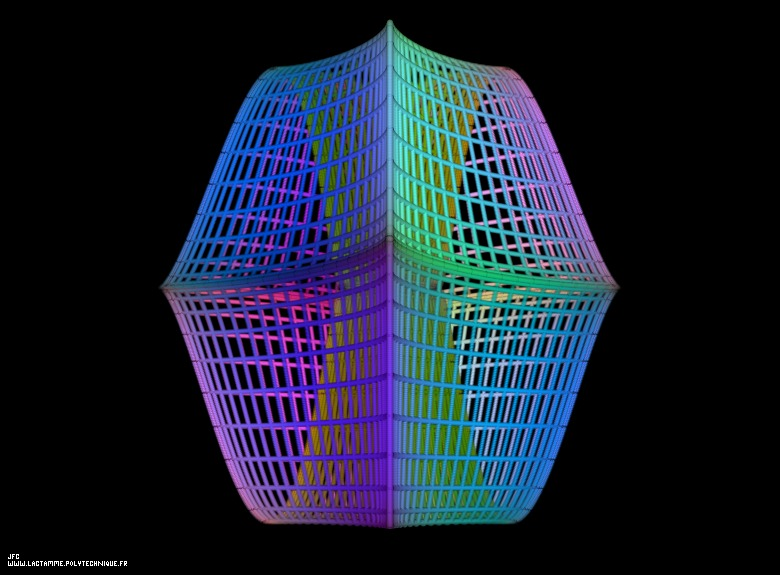The third 'power' of a torus defined by means of three bidimensional fields [La troisième 'puissance' d'un tore définie à l'aide de trois champs bidimensionnels].

Many surfaces -bidimensional manifolds- in a tridimensional space can be defined using a set of three equations:
```                    X = F (u,v)
x
```
```                    Y = F (u,v)
y
```
```                    Z = F (u,v)
z
```
with:
```                    u E [U   ,U   ]
min  max
```
```                    v E [V   ,V   ]
min  max
```
[Umin,Umax]x[Vmin,Vmax] then defined a bidimensional rectangular domain D.
```                       v ^
|
V    |...... ---------------------------
max |      |+++++++++++++++++++++++++++|
|      |+++++++++++++++++++++++++++|
|      |+++++++++++++++++++++++++++|
|      |+++++++++++++++++++++++++++|
|      |+++++++++++++++++++++++++++|
|      |+++++++++++++++++++++++++++|
|      |+++++++++++++++++++++++++++|
|      |+++++++++++++++++++++++++++|
|      |+++++++++++++++++++++++++++|
V    |...... ---------------------------
min |      :                           :
|      :                           :
O------------------------------------------------->
U                           U              u
min                         max
```

If D is sampled by means of a bidimensional rectangular grid (made of Nu.Nv points), the three {X,Y,Z} coordinates can be defined by means of three rectangular matrices:
```                    X = M (i,j)
x
```
```                    Y = M (i,j)
y
```
```                    Z = M (i,j)
z
```
with:
```                    i = f(u,U   ,U   ,N )
min  max  u
```
```                    j = g(v,V   ,V   ,N )
min  max  v
```
where 'f' and 'g' denote two obvious linear functions...

[for more information about this process]
[pour plus d'informations sur ce processus]

For the third 'power' of a torus, the three {X,Y,Z} fields/matrices are as follows:

(CMAP28 WWW site: this page was created on 02/09/2015 and last updated on 01/26/2019 11:54:18 -CET-)

[See all related pictures (including this one) [Voir toutes les images associées (incluant celle-ci)]]

[Go back to AVirtualSpaceTimeTravelMachine [Retour à AVirtualSpaceTimeTravelMachine]]
[The Y2K bug [Le bug de l'an 2000]]

[Site Map, Help and Search [Plan du Site, Aide et Recherche]]
[Mail [Courrier]]
[About Pictures and Animations [A Propos des Images et des Animations]]